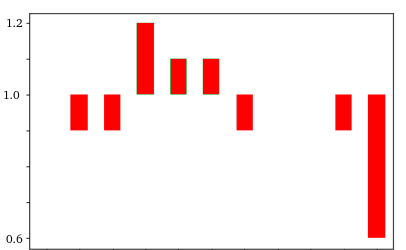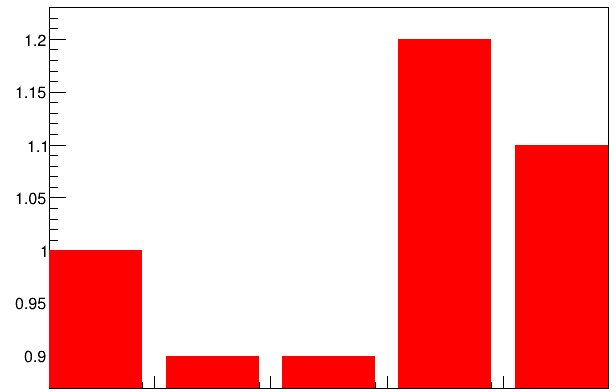# Bar graph. Can vertical bars start at one instead of zero?

Dear Rooters,

I would like to produce a graph like this screenshot:This could be a minimal working example to produce a similar graph

``````std::vector<double> x_v = {1.,2.,3.,4.,5.}
std::vector<double> y_v = {1.0, 0.9, 0.9, 1.2, 1.1 }
gr = new TGraph( x_v.size(), x_v.data(), y_v.data())
gr->SetFillColor(kRed)
gr->Draw("AB")

``````

This code results in this plot:I would like the bars to start at 1 instead of zero, as in case of the first image. Is there a simply way to do it? I would prefer to avoid “tricking the data” (subtracting 1 to each element in y_v), because I would like also to plot it in logarithmic scale.

I spent some time looking for similar problems, but I found nothing. Sorry if this question was already answered.

Cheers,
atd

ROOT Version: 6.20
Platform: Centos 8
Compiler: gcc 8.3

Hi,

the following code gives exactly what you want:

``````void atd()
{
std::vector<double> x_v = { 1,   2,  3,   4,   5,    6,    7,   8,  9,   10};
std::vector<double> ex_v = {.2, .2,  .2,  .2,  .2,   .2,   .2,  .2, .2,  .2};
std::vector<double> y_v = { -1, .95, .95, 1.1, 1.05, 1.05, .95, -1, .95, .8};
std::vector<double> ey_v = {.0, .05, .05, .1,  .05,  .05,  .05, .0, .05, .2};

TGraphErrors * gr = new TGraphErrors( x_v.size(), x_v.data(), y_v.data(), ex_v.data(), ey_v.data());
gr->SetFillColor(kRed);
gr->SetMinimum(0.55); gr->SetMaximum(1.25);
gr->Draw("AE2");
}
``````

1 Like

This topic was automatically closed 14 days after the last reply. New replies are no longer allowed.